# Sampling Methods and the Central Limit Theorem Chapter

• Slides: 12GOALS 1. 2. 3. 4. 5. 8 -2 Explain why a sample is the only feasible way to learn about a population. Describe methods to select a sample. Define and construct a sampling distribution of the sample mean. Explain the central limit theorem. Use the central limit theorem to find probabilities of selecting possible sample means from a specified population.Probability Sampling Why Sample the Population? 1. To contact the whole population would be time consuming. 2. The cost of studying all the items in a population may be prohibitive. 3. The physical impossibility of checking all items in the population. 4. The destructive nature of some tests. 5. The sample results are adequate. What is a Probability Sample? A probability sample is a sample selected such that each item or person in the population being studied has a known likelihood of being included in the sample. Four Most Commonly Used Probability Sampling Methods 1. Simple Random Sample 2. Systematic Random Sampling 3. Stratified Random Sampling 4. Cluster Sampling 8 -3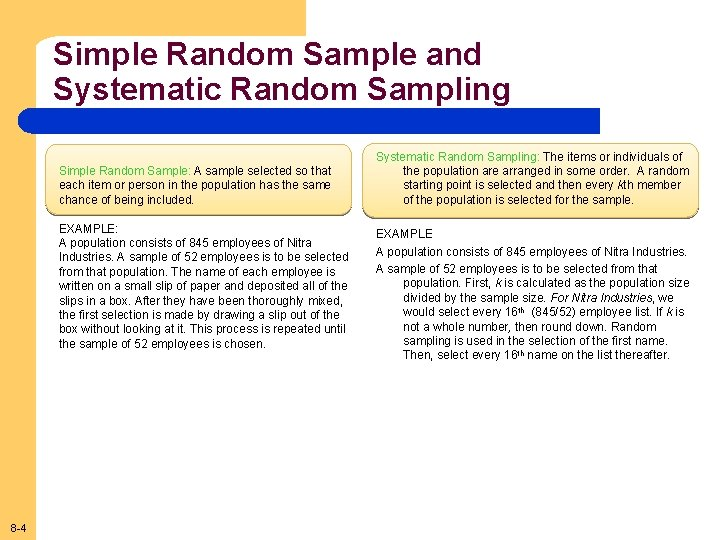Simple Random Sample and Systematic Random Sampling Simple Random Sample: A sample selected so that each item or person in the population has the same chance of being included. EXAMPLE: A population consists of 845 employees of Nitra Industries. A sample of 52 employees is to be selected from that population. The name of each employee is written on a small slip of paper and deposited all of the slips in a box. After they have been thoroughly mixed, the first selection is made by drawing a slip out of the box without looking at it. This process is repeated until the sample of 52 employees is chosen. 8 -4 Systematic Random Sampling: The items or individuals of the population are arranged in some order. A random starting point is selected and then every kth member of the population is selected for the sample. EXAMPLE A population consists of 845 employees of Nitra Industries. A sample of 52 employees is to be selected from that population. First, k is calculated as the population size divided by the sample size. For Nitra Industries, we would select every 16 th (845/52) employee list. If k is not a whole number, then round down. Random sampling is used in the selection of the first name. Then, select every 16 th name on the list thereafter.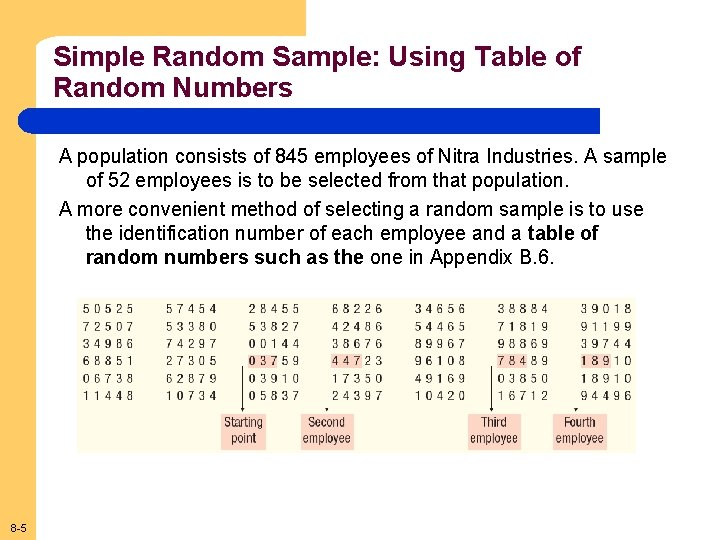Simple Random Sample: Using Table of Random Numbers A population consists of 845 employees of Nitra Industries. A sample of 52 employees is to be selected from that population. A more convenient method of selecting a random sample is to use the identification number of each employee and a table of random numbers such as the one in Appendix B. 6. 8 -5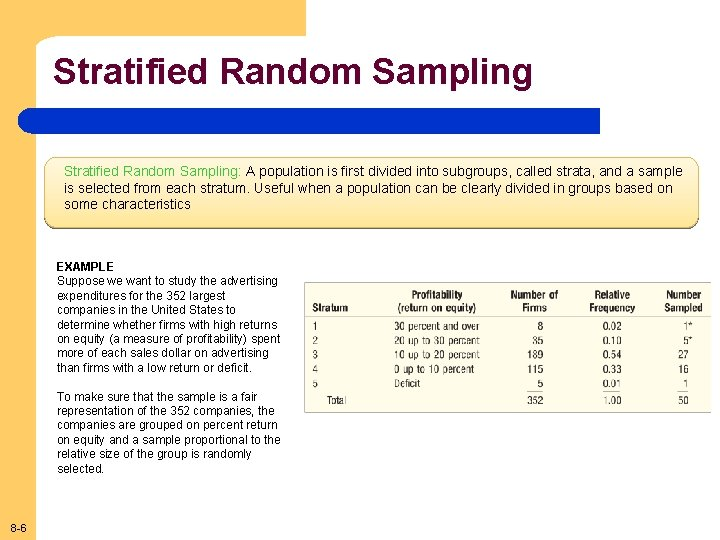Stratified Random Sampling: A population is first divided into subgroups, called strata, and a sample is selected from each stratum. Useful when a population can be clearly divided in groups based on some characteristics EXAMPLE Suppose we want to study the advertising expenditures for the 352 largest companies in the United States to determine whether firms with high returns on equity (a measure of profitability) spent more of each sales dollar on advertising than firms with a low return or deficit. To make sure that the sample is a fair representation of the 352 companies, the companies are grouped on percent return on equity and a sample proportional to the relative size of the group is randomly selected. 8 -6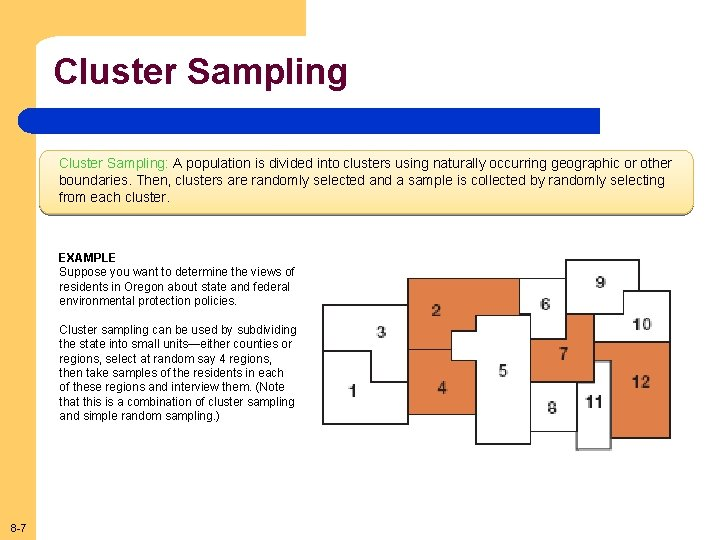Cluster Sampling: A population is divided into clusters using naturally occurring geographic or other boundaries. Then, clusters are randomly selected and a sample is collected by randomly selecting from each cluster. EXAMPLE Suppose you want to determine the views of residents in Oregon about state and federal environmental protection policies. Cluster sampling can be used by subdividing the state into small units—either counties or regions, select at random say 4 regions, then take samples of the residents in each of these regions and interview them. (Note that this is a combination of cluster sampling and simple random sampling. ) 8 -7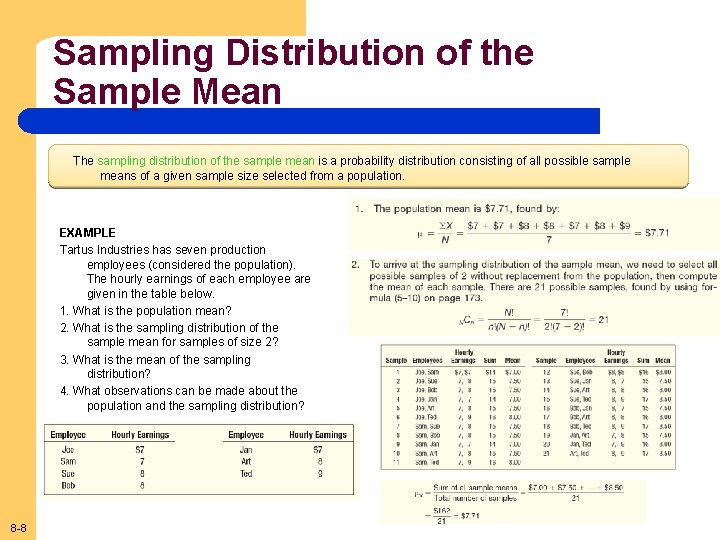Sampling Distribution of the Sample Mean The sampling distribution of the sample mean is a probability distribution consisting of all possible sample means of a given sample size selected from a population. EXAMPLE Tartus Industries has seven production employees (considered the population). The hourly earnings of each employee are given in the table below. 1. What is the population mean? 2. What is the sampling distribution of the sample mean for samples of size 2? 3. What is the mean of the sampling distribution? 4. What observations can be made about the population and the sampling distribution? 8 -8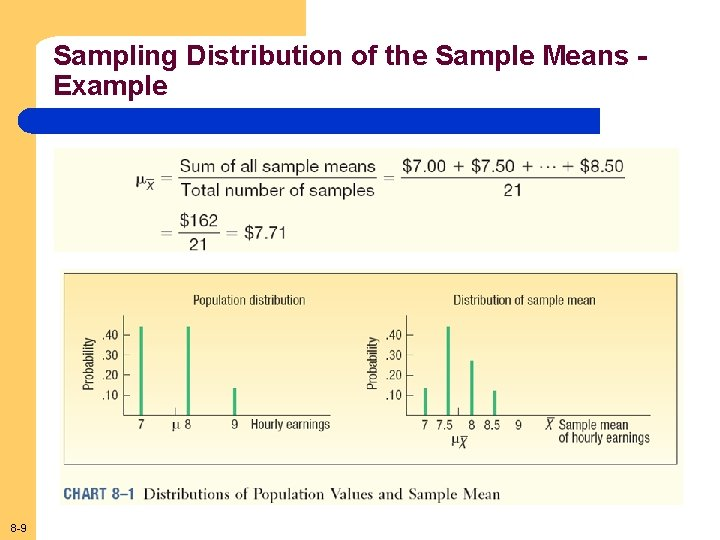Sampling Distribution of the Sample Means Example 8 -9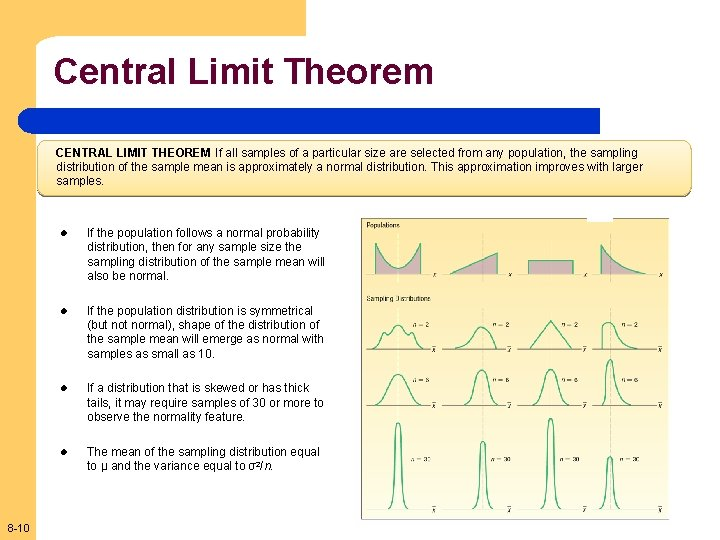Central Limit Theorem CENTRAL LIMIT THEOREM If all samples of a particular size are selected from any population, the sampling distribution of the sample mean is approximately a normal distribution. This approximation improves with larger samples. 8 -10 l If the population follows a normal probability distribution, then for any sample size the sampling distribution of the sample mean will also be normal. l If the population distribution is symmetrical (but normal), shape of the distribution of the sample mean will emerge as normal with samples as small as 10. l If a distribution that is skewed or has thick tails, it may require samples of 30 or more to observe the normality feature. l The mean of the sampling distribution equal to μ and the variance equal to σ2/n.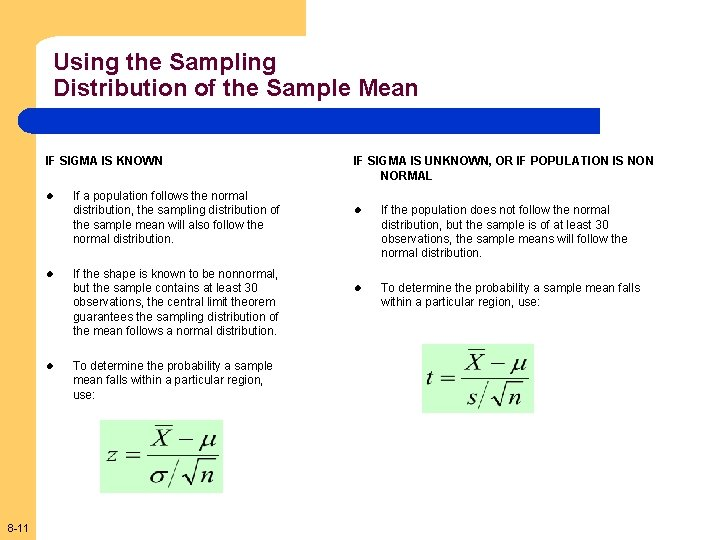Using the Sampling Distribution of the Sample Mean IF SIGMA IS KNOWN l l l 8 -11 If a population follows the normal distribution, the sampling distribution of the sample mean will also follow the normal distribution. If the shape is known to be nonnormal, but the sample contains at least 30 observations, the central limit theorem guarantees the sampling distribution of the mean follows a normal distribution. To determine the probability a sample mean falls within a particular region, use: IF SIGMA IS UNKNOWN, OR IF POPULATION IS NON NORMAL l If the population does not follow the normal distribution, but the sample is of at least 30 observations, the sample means will follow the normal distribution. l To determine the probability a sample mean falls within a particular region, use:Using the Sampling Distribution of the Sample Mean (Sigma Known) - Example EXAMPLE The Quality Assurance Department for Cola, Inc. , maintains records regarding the amount of cola in its Jumbo bottle. The actual amount of cola in each bottle is critical, but varies a small amount from one bottle to the next. Cola, Inc. , does not wish to underfill the bottles. On the other hand, it cannot overfill each bottle. Its records indicate that the amount of cola follows the normal probability distribution. The mean amount per bottle is 31. 2 ounces and the population standard deviation is 0. 4 ounces. Solution: Step 1: Find the z-values corresponding to the sample mean of 31. 38 Step 2: Find the probability of observing a Z equal to or greater than 1. 80 At 8 A. M. today the quality technician randomly selected 16 bottles from the filling line. The mean amount of cola contained in the bottles is 31. 38 ounces. Is this an unlikely result? Is it likely the process is putting too much soda in the bottles? To put it another way, is the sampling error of 0. 18 ounces unusual? 8 -12 Conclusion: It is unlikely, less than a 4 percent chance, we could select a sample of 16 observations from a normal population with a mean of 31. 2 ounces and a population standard deviation of 0. 4 ounces and find the sample mean equal to or greater than 31. 38 ounces. The process is putting too much cola in the bottles.